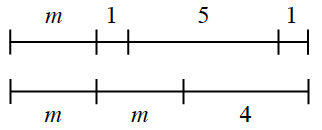### Home > CC2 > Chapter Ch6 > Lesson 6.2.5 > Problem6-109

6-109.The diagram at right represents an acrobat’s sequence on a tightrope, where m represents the distance in feet that she covers each time she does a leap.

1. How long is each of her leaps? How can you tell?

The diagram reveals that $m+1+5+1$ is the same distance as $m+m+4$.
Use these distances to create an equation that will allow you to solve for $m$.

2. Write and solve an equation to find the length of each leap.

See part (a).

$m+1+5+1=m+m+4$

Simplify the equation.
$7+m=4+2m$

Solve for $m$.

$m=3$ feet

3. How long is the tightrope?

Substitute $m$ in the equation with the answer you found in part (b).

$10$ feet